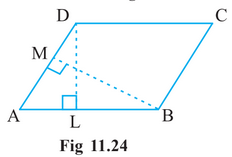Q

# DL and BM are the heights on sides AB and AD respectively, of parallelogram ABCD (Fig 11.24). If the area of the parallelogram is 1470 cm^2.

Q. 6.     and are the heights on sides and respectively, of parallelogram (Fig 11.24). If the area of the parallelogram is

and  find the length of andViews
R

We know that

Area of parallelogram

Here,

Base of parallelogram(AB) = 35 cm

and

Height of parallelogram(DL) = h cm

Similarly,

Area is also given by

Therefore, the value of BM and DL are  is  30cm and 42cm respectively

Exams
Articles
Questions